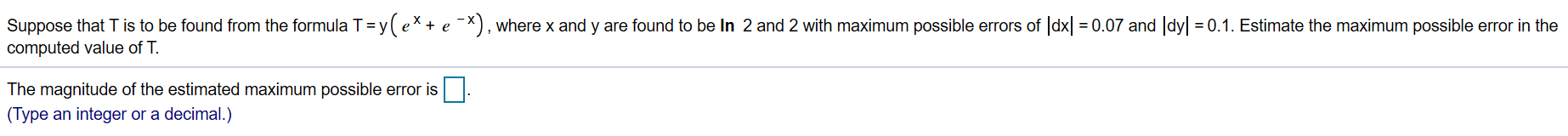# Suppose that T is to be found from the formula T = y( e* + e ^X), where x and y are found to be In 2 and 2 with maximum possible errors of dx 0.07 and dy 0.1. Estimate the maximum possible error in thecomputed value of TThe magnitude of the estimated maximum possible error is(Type an integer or a decimal.)

Question

Estimate the maximum possible error in the computed value of T (Included in the image).help_outlineImage TranscriptioncloseSuppose that T is to be found from the formula T = y( e* + e ^X), where x and y are found to be In 2 and 2 with maximum possible errors of dx 0.07 and dy 0.1. Estimate the maximum possible error in the computed value of T The magnitude of the estimated maximum possible error is (Type an integer or a decimal.) fullscreen
check_circle

Step 1

Differentiate both sides of...

### Want to see the full answer?

See Solution

#### Want to see this answer and more?

Solutions are written by subject experts who are available 24/7. Questions are typically answered within 1 hour.*

See Solution
*Response times may vary by subject and question.
Tagged in

### Other